SYLLABUS  Previous: 5 MONTE-CARLO METHOD  Up: 5 MONTE-CARLO METHOD  Next: 5.2 Stochastic theory

## 5.1 Monte Carlo integration

Slide : [ integration || VIDEO login]

The most common use of Monte Carlo methods is the evaluation of multi-dimensional integrals . Consider first the approximation of an integral obtained from the trapezoidal rule (3.3#eq.1)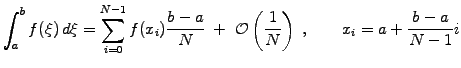(1)

Instead of a uniform sampling, imagine an evaluation where the positions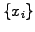are random numbers uniformly distributed in the interval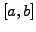; this yields a Monte Carlo integration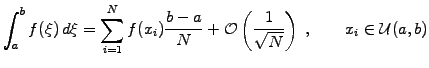(2)

The convergence rate is of course lower than from a uniform sampling (5.1#eq.1); the strength however appears for integrals in higher dimensions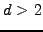, where the Monte Carlo error scales as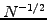irrespective of the number of dimensions - instead of the scaling in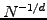that is achieved when using a uniform mesh.